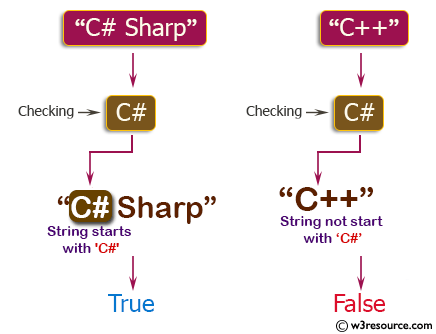﻿ C++ Exercises: Check if a given string starts with 'C#' or not - w3resource# C++ Exercises: Check if a given string starts with 'C#' or not

## C++ Basic Algorithm: Exercise-12 with Solution

Write a C++ program to check if a given string starts with 'C#' or not.

Sample Solution:

C++ Code :

``````#include <iostream>

using namespace std;

bool test(string str)
{
return (str.length() < 3 && str=="C#") || (str.substr(0,2)=="C#" && str == ' ');
}

int main()
{
cout << test("C# Sharp") << endl;
cout << test("C#") << endl;
cout << test("C++") << endl;
return 0;
}
``````

Sample Output:

```1
1
0
```

Pictorial Presentation:Flowchart:C++ Code Editor:

What is the difficulty level of this exercise?

﻿

```std::priority_queue<int, std::vector<int>, std::greater<int> > my_min_heap;